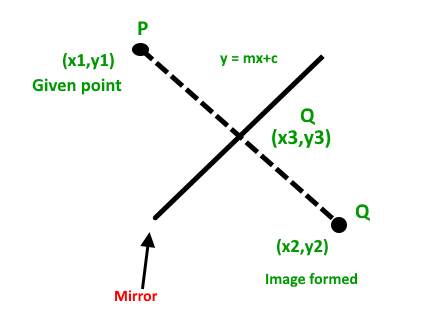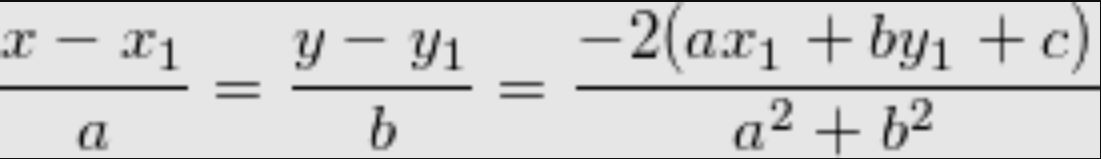Open In App
Related Articles

# Find mirror image of a point in 2-D plane

Given a point P in 2-D plane and equation of mirror, the task is to find the image of that point Q formed due to the mirror.
Equation of mirror is in form ax + by + c = 0
Examples:

Input : P = (1, 0), a = -1, b = 1, c = 0
Output : Q = (0, 1)

Input : P = (3, 3), a = 0, b = 1, c = -2
Output : Q = (3, 1)

## Solution :```Let coordinate of P(given point) be (x1, y1)
Let coordinate of Q(image point) be (x2, y2)
Let coordinate of R(point on mirror) be (x3, y3)```

Since object and image are equidistant from mirror, R must be middle point of P and Q
Since equation of mirror is given to be: ax + by + c = 0. Equation of line passing through P and Q is perpendicular to mirror. Therefore equation of line passing through P and Q becomes ay – bx + d = 0, Also P passes through line passing through P and Q, so we put coordinate of P in above equation,
a*y1 – b*x1 + d = 0
d = b*x1 – a*y1
Also R is intersection of mirror and line passing through P and Q. So we find solution of
ax + by + c = 0
ay -bx + d = 0
Since a, b, c, d all are known we can find x and y here. Since coordinates of R is known now ie.. x3, y3 are known now.
Since R is middle point of PQ,
(x3, y3) = ((x1+x2)/2, (y1+y2)/2)
Since x1, y1, x3, y3 are known, we get below equation where (x, y) are coordinates of Q(image point)We use above formula to find mirror image of point P(x1, y1) with respect to mirror of equation ax + by + c

## C++

 `// C++ code to find mirror image``#include ``using` `namespace` `std;` `// C++ function which finds coordinates``// of mirror image.``// This function return a pair of double``pair<``double``, ``double``> mirrorImage(``    ``double` `a, ``double` `b, ``double` `c,``    ``double` `x1, ``double` `y1)``{``    ``double` `temp = -2 * (a * x1 + b * y1 + c) /``                              ``(a * a + b * b);``    ``double` `x = temp * a + x1;``    ``double` `y = temp * b + y1;``    ``return` `make_pair(x, y);``}` `// Driver code to test above function``int` `main()``{``    ``double` `a = -1.0;``    ``double` `b = 1.0;``    ``double` `c = 0.0;``    ``double` `x1 = 1.0;``    ``double` `y1 = 0.0;` `    ``pair<``double``, ``double``> image = mirrorImage(a, b, c, x1, y1);``    ``cout << ``"Image of point ("` `<< x1 << ``", "` `<< y1 << ``") "``;``    ``cout << ``"by mirror ("` `<< a << ``")x + ("` `<< b``         ``<< ``")y + ("` `<< c << ``") = 0, is :"``;``    ``cout << ``"("` `<< image.first << ``", "` `<< image.second``         ``<< ``")"` `<< endl;` `    ``return` `0;``}`

## Java

 `// Java code to find mirror image``class` `GFG``{``static` `class` `pair``{``    ``double` `first, second;``    ``public` `pair(``double` `first, ``double` `second)``    ``{``        ``this``.first = first;``        ``this``.second = second;``    ``}``}` `// function which finds coordinates``// of mirror image.``// This function return a pair of double``static` `pair mirrorImage(``double` `a, ``double` `b,``                        ``double` `c, ``double` `x1,``                        ``double` `y1)``{``    ``double` `temp = -``2` `* (a * x1 + b * y1 + c) /``                       ``(a * a + b * b);``    ``double` `x = temp * a + x1;``    ``double` `y = temp * b + y1;``    ``return` `new` `pair(x, y);``}` `// Driver code``public` `static` `void` `main(String []args)``{``    ``double` `a = -``1.0``;``    ``double` `b = ``1.0``;``    ``double` `c = ``0.0``;``    ``double` `x1 = ``1.0``;``    ``double` `y1 = ``0.0``;` `    ``pair image = mirrorImage(a, b, c, x1, y1);``    ``System.out.print(``"Image of point ("` `+ x1 +``                                   ``", "` `+ y1 + ``") "``);``    ``System.out.print(``"by mirror ("` `+ a +``                          ``")x + ("` `+ b +``                          ``")y + ("` `+ c + ``") = 0, is :"``);``    ``System.out.println(``"("` `+ image.first +``                       ``", "` `+ image.second + ``")"``);``}``}` `// This code is contributed by 29AjayKumar`

## Python 3

 `# Python 3 code to find mirror image` `# Python function which finds coordinates``# of mirror image.``# This function return a pair of double``def` `mirrorImage( a, b, c, x1, y1):``    ``temp ``=` `-``2` `*` `(a ``*` `x1 ``+` `b ``*` `y1 ``+` `c) ``/``(a ``*` `a ``+` `b ``*` `b)``    ``x ``=` `temp ``*` `a ``+` `x1``    ``y ``=` `temp ``*` `b ``+` `y1``    ``return` `(x, y)` `# Driver code to test above function``a ``=` `-``1.0``b ``=` `1.0``c ``=` `0.0``x1 ``=` `1.0``y1 ``=` `0.0` `x, y ``=` `mirrorImage(a, b, c, x1, y1);``print``(``"Image of point ("` `+` `str` `(x1) ``+` `", "` `+` `str``( y1) ``+` `") "``)``print``(``"by mirror ("` `+` `str` `(a) ``+` `")x + ("` `+` `str``( b) ``+` `")y + ("` `+``str``(c) ``+` `") = 0, is :"``)``print``( ``"("` `+` `str``(x) ``+` `", "` `+` `str``(y) ``+` `")"` `)` `# This code is contributed by ApurvaRaj`

## C#

 `// C# code to find mirror image``using` `System;` `class` `GFG``{``class` `pair``{``    ``public` `double` `first, second;``    ``public` `pair(``double` `first, ``double` `second)``    ``{``        ``this``.first = first;``        ``this``.second = second;``    ``}``}` `// function which finds coordinates``// of mirror image.``// This function return a pair of double``static` `pair mirrorImage(``double` `a, ``double` `b,``                        ``double` `c, ``double` `x1,``                        ``double` `y1)``{``    ``double` `temp = -2 * (a * x1 + b * y1 + c) /``                       ``(a * a + b * b);``    ``double` `x = temp * a + x1;``    ``double` `y = temp * b + y1;``    ``return` `new` `pair(x, y);``}` `// Driver code``public` `static` `void` `Main(String []args)``{``    ``double` `a = -1.0;``    ``double` `b = 1.0;``    ``double` `c = 0.0;``    ``double` `x1 = 1.0;``    ``double` `y1 = 0.0;` `    ``pair image = mirrorImage(a, b, c, x1, y1);``    ``Console.Write(``"Image of point ("` `+ x1 +``                                ``", "` `+ y1 + ``") "``);``    ``Console.Write(``"by mirror ("` `+ a +``                       ``")x + ("` `+ b +``                       ``")y + ("` `+ c + ``") = 0, is :"``);``    ``Console.WriteLine(``"("` `+ image.first +``                     ``", "` `+ image.second + ``")"``);``}``}` `// This code is contributed by PrinciRaj1992`

## Javascript

 ``

Output

```Image of point (1, 0) by mirror (-1)x + (1)y + (0) = 0, is :(0, 1)
```

Time Complexity: O(1)
Auxiliary Space: O(1)
This article is contributed by Pratik Chhajer. If you like GeeksforGeeks and would like to contribute, you can also write an article using write.geeksforgeeks.org or mail your article to review-team@geeksforgeeks.org. See your article appearing on the GeeksforGeeks main page and help other Geeks.